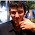Please see my updated post:Convert RGB(A) output to Hex Color

My current project uses Farbtastic which is a fantastic jQuery plugin which allows users to visually choose a color for an element. The problem I ran into was loading a default color from the element:

jQuery returns the element color in RGB format
document.write( \$('#myElement').css('background-color') );
// outputs: rgb(34, 34, 34)

but Farbtastic uses the hex color format, just like the CSS.
#myElement { background-color: #222222 }
I found this really nice script (by R0bb13) which converts RGB to hex. This script requires the input variable to be in an array "[34,34,34]". In order to make the script use an RGB format "rgb(34,34,34)", I had to add one line to the script to remove the "rgb" and parenthesis and then convert (split) the result into an array...

Update #2 (allows entering an rgba value - opacity is ignored)

Updated (removing internal function):
//Function to convert hex format to a rgb color
function rgb2hex(orig){
var rgb = orig.replace(/\s/g,'').match(/^rgba?\((\d+),(\d+),(\d+)/i);
return (rgb && rgb.length === 4) ? "#" +
("0" + parseInt(rgb,10).toString(16)).slice(-2) +
("0" + parseInt(rgb,10).toString(16)).slice(-2) +
("0" + parseInt(rgb,10).toString(16)).slice(-2) : orig;
}
Here is an example of how to use this code with jQuery:
document.write( rgb2hex(\$('#myElement').css('background-color')) );
// outputs: #222222
Here is the original code from this post (not nearly as efficient as the updated script):
//Function to convert hex format to a rgb color
function rgb2hex(rgb) {
var hexDigits = ["0","1","2","3","4","5","6","7","8","9","a","b","c","d","e","f"];
rgb = rgb.match(/^rgb\((\d+),\s*(\d+),\s*(\d+)\)\$/);
function hex(x) {
return isNaN(x) ? "00" : hexDigits[(x - x % 16) / 16] + hexDigits[x % 16];
}
return "#" + hex(rgb) + hex(rgb) + hex(rgb);
}

1.2.thank you so much :)

3.I've added an alternative method that doesn't require an array, *smack head* just use the built in functions (duh LOL)

4.Nice one motty, great post! Would you consider writing some jQuery posts for my blog? http://jquery4u.com obviously will be some incentives. Cheers. Sam

5.Just for info !

This script breaks awfully when encountering a css3 rgba string !

rgba(0,0,0,0)

http://www.zenelements.com/blog/css3-rgb-rgba-color-opacity/

6.Thanks Kim! I modified the script a bit, here is a demo.

7.yeeesssss, excellent and simple

8.Thank you! Worked like a charm here!

9.